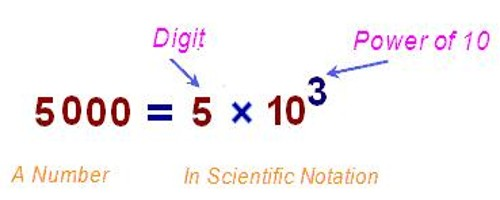Convert Scientific Notation to Decimal Numbers

Scientific notation (SN), also referred to as exponential notation, is a system developed to abbreviate numbers having an extreme number of digits and or decimal places. When working with very large or very small numbers, scientists, mathematicians, and engineers often use scientific notation to express those quantities. Scientific notation uses exponential notation. The following are examples of scientific notation.

To change a number from scientific notation to standard form, move the decimal point to the left (if the exponent of ten is a negative number), or to the right (if the exponent is positive). You should move the point as many times as the exponent indicates. Do not write the power of ten anymore.Scientific notation consists of a coefficient (here 5.14) multiplied by 10 raised to an exponent (here 5). To convert to a real number, start with the base and multiply by 5 tens like this: 5.14 × 10 × 10 × 10 × 10 × 10 = 514000.0. Multiplying by tens is easy: one simply moves the decimal point in the base (5.14) 5 places to the right, adding extra zeroes as needed.

5.14 × 105

= 51.4 × 104

= 514.0 × 103

= 5140.0 × 102

= 51400.0 × 101

= 514000.0 × 100

= 514000.0

Or, to convert the scientific notation 1.23 x 10-11 to regular notation you simply move the decimal point 11 places to the left, as indicated by the exponent -11.

1.23 x 10-11 converts to the decimal number 0.0000000000123

Scientific notation is used to express very large or very small numbers. A number in scientific notation is written as the product of a number (integer or decimal) and a power of 10. The number has one digit to the left of the decimal point. The power of ten indicates how many places the decimal point was moved.

The number 6.5×10-7 written in decimal format would be 0.00000065 because the decimal point was moved 7 places to the left to form the decimal 0.00000065.

Information Source: